# Vehicular Emissions INventories (VEIN)### What is new?

• Coming soon: Projects for Latin America, Europe and USA

### TODO

• get_project with Europe and Chinese EF
• Update ef_ldv* and ef_hdv* to EEA2019
• Include speed functions with Fortran
• Include CB6
• See issues GitHub and GitLab

### System requirements

vein imports functions from spatial packages listed below. In order to install these packages, firstly the user must install the requirements mentioned here.

### Installation

#### CRAN

VEIN can be installed via CRAN or github

install.packages("vein")

#### GitHub

remotes::install_github("atmoschem/vein")

or if you have a 32 bits machine

install_github("atmoschem/vein",
INSTALL_opts = "--no-multiarch")

#### demo

Then, if you want, run the demo

library(vein)
demo(VEIN)

## Approaches

### 1. get a project (easier)

At the moment, most of the projects covers Brazilian regions, but I will include China, Europe or USA approaches as soon as I can.

Use the function get_project and read the documentation.

library(vein)
?get_project
get_project(directory = "awesome_city")

The structure of the new directory “awesome_city” is:

awesome_city
├── config
│   ├── clean.R
│   ├── config.R
│   ├── inventory.xlsx
│   └── packages.R
├── main.R
├── main.Rproj
├── network
│   ├── net.gpkg
│   └── net.rds
├── scripts
│   ├── evaporatives.R
│   ├── exhaust.R
│   ├── fuel_eval.R
│   ├── net.R
│   ├── plots.R
│   ├── post.R
│   ├── traffic.R
│   └── wrf.R
└── wrf
└── wrfinput_d02

You have to open the file main.Rproj with Rstudio and then open and run main.R

To run main.R you will need these extra packages:

• ggplot2
• eixport (If you plan to generate WRF Chem emissions file)

If you do not have them already, you can install:

install.packages(c("ggplot2", "readxl", "eixport"))

## Too complicated? Watch a YouTube

English

Portuguese

Spanish coming soon!

### 2. Use inventory (a bit not so easy)

?inventory

#### 1) Examples with traffic data:

1. If you know the distribution of the vehicles by age of use , use: my_age
2. If you know the sales of vehicles or better the registry of new vehicles, use age to apply a survival function.
3. If you know the theoretical shape of the circulating fleet and you can use age_ldv, age_hdv or age_moto. For instance, you dont know the sales or registry of vehicles, but somehow you know the shape of this curve.
4. You can use/merge/transform/adapt any of these functions.
library(vein)
data("net")
PC_E25_1400 <- age_ldv(
x = net$ldv ) plot( PC_E25_1400 ) #> Weighted mean = 11.17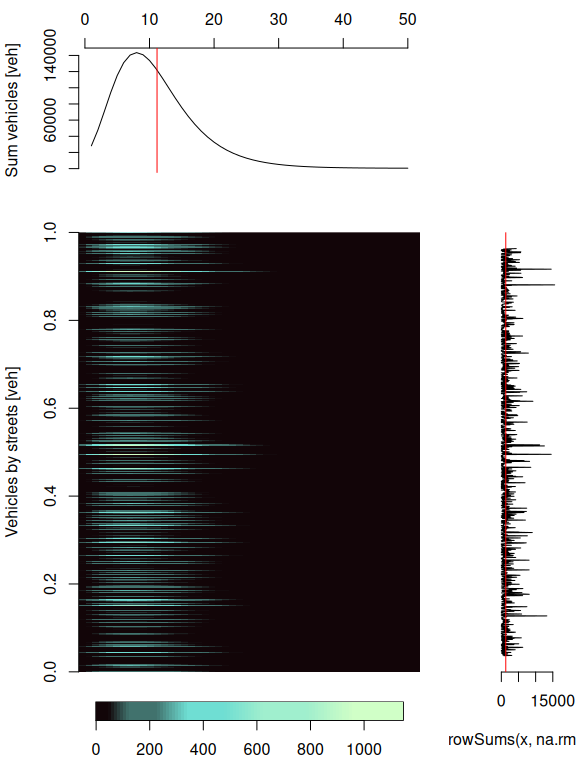If you want to know the vehicles per street and by age of use, just add the net. Age functions now returns ‘sf’ objects if the net argument is present. PC_E25_1400net <- age_ldv( x = net$ldv,
net = net
)
plot(
PC_E25_1400net,
key.pos = 4,
pal = cptcity::cpt(
colorRampPalette = T,
rev = T
)
)
#> Warning: plotting the first 9 out of 50 attributes; use max.plot = 50 to plot
#> all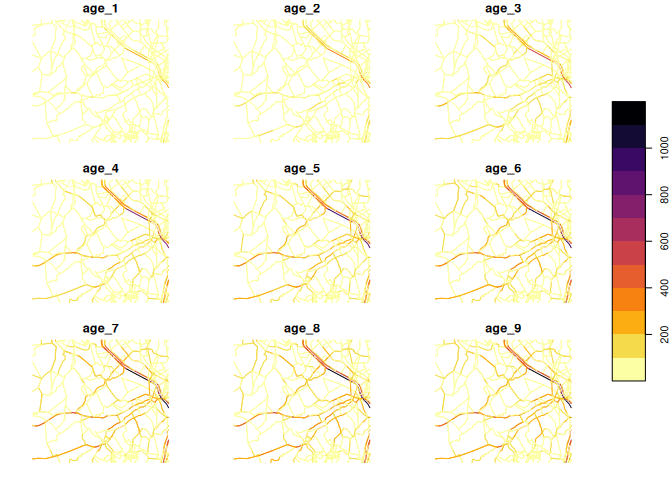temporal factors and netspeed

data("net")
data("pc_profile")
pc_week <- temp_fact(
net$ldv + net$hdv,
pc_profile
)
dfspeed <- netspeed(
q = pc_week,
ps = net$ps, ffs = net$ffs,
cap = net$capacity, lkm = net$lkm,
alpha = 1.5
)
plot(dfspeed)
#> Weighted mean =  44.16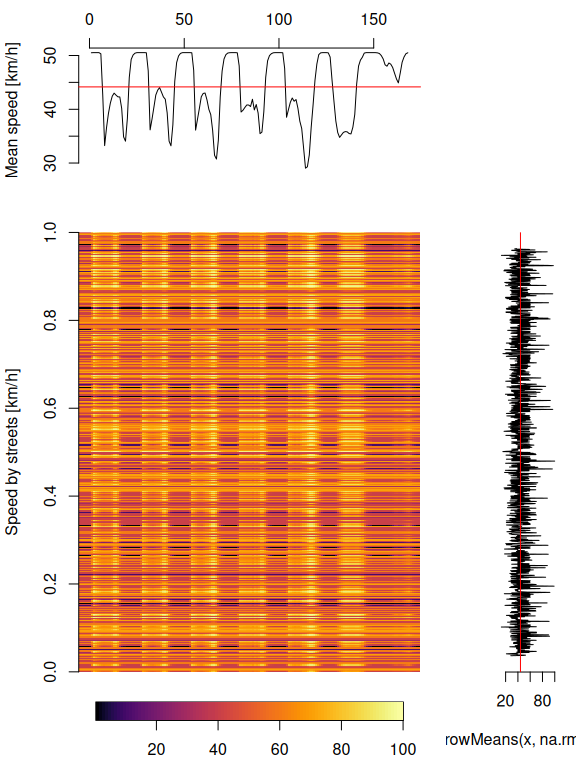If you want ot check the speed at different hours by street, just add net:

dfspeednet <- netspeed(
q = pc_week,
ps = net$ps, ffs = net$ffs,
cap = net$capacity, lkm = net$lkm,
alpha = 1.5,
net = net
)
plot(
dfspeednet[, c("S1", "S9")],
key.pos = 4,
pal = cptcity::cpt(colorRampPalette = T,
rev = T),
axes = T
)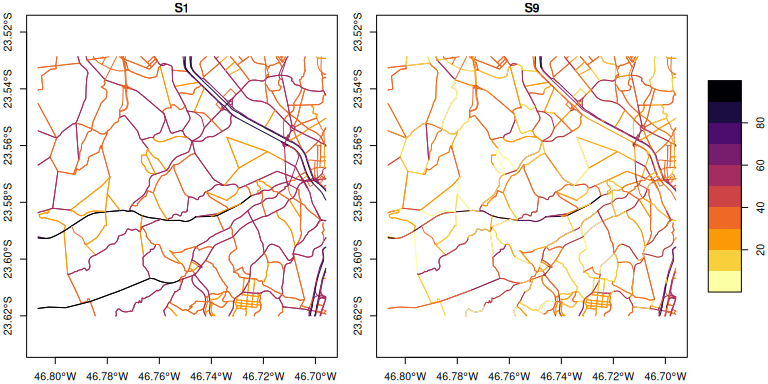#### 2) Emission Factors

V <- 0:150
ef1 <- ef_ldv_speed(
v = "PC",
t = "4S",
cc = "<=1400",
f = "G",
eu = "PRE",
p = "CO"
)
efs <- EmissionFactors(ef1(1:150))
plot(
Speed(1:150),
efs,
xlab = "speed[km/h]",
type = "b",
pch = 16
)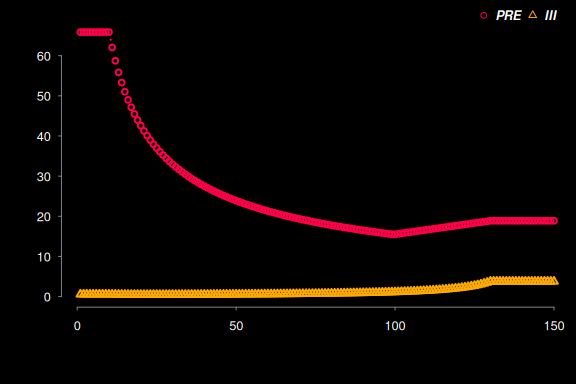#### 3) Estimation of emissions

euro <- c(
rep("V", 5),
rep("IV", 5),
rep("III", 5),
rep("II", 5),
rep("I", 5),
rep("PRE", 15)
)
lef <- lapply(1:40, function(i) {
ef_ldv_speed(
v = "PC",
t = "4S",
cc = "<=1400",
f = "G",
eu = euro[i],
p = "CO",
show.equation = FALSE
)
})
E_CO <- emis(
veh = PC_E25_1400,
lkm = net\$lkm,
ef = lef,
speed = dfspeed,
profile = pc_profile
)

#### 4) Post Emissions

• emis_post
• When the argument by = “veh” the emissions are aggregated by age and hour.
• When the argument by = “streets_wide”, aggregated the emissions by street. In this cae, if you add the argument net with the respective streets, it returns an spatial net with the hourly emissions.
E_CO_DF <- emis_post(
arra = E_CO,
veh = "PC",
size = "<1400",
fuel = "G",
pollutant = "CO",
by = "veh",
type_emi = "exhaust"
)
E_CO_STREETS <- emis_post(
arra = E_CO,
pollutant = "CO",
by = "streets",
net = net
)
plot(
E_CO_STREETS[, c("V1", "V9")],
key.pos = 4,
pal = cptcity::cpt(colorRampPalette = T,
rev = T),
axes = T)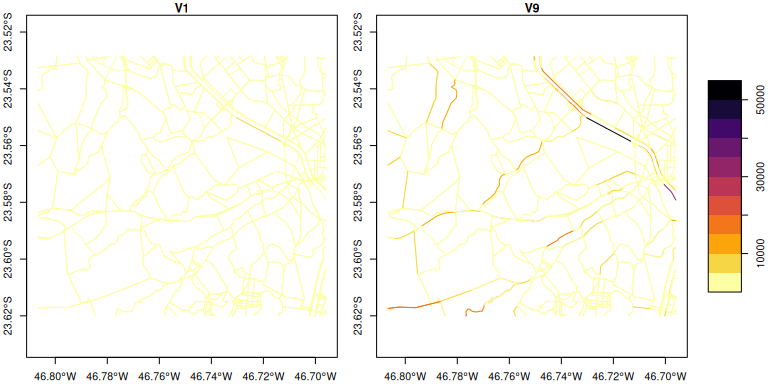#### Grids

1. Create a grid using make_grid.The spobj is the spatial net. The size of the grid has the size of the net. You have to specify the grid spacing.
2. Create a grid using a path to wrfinput file instead a net. The grid will have the size of the wrf_input. You don’t have to specify the grid spacing.
data(net)
E_CO_STREETSnet <- emis_post(
arra = E_CO,
pollutant = "CO",
by = "streets_wide",
net = net
)
g <- make_grid(
spobj = net,
width = 1/102.47
)
#> Number of lon points: 12
#> Number of lat points: 10
E_CO_g <- emis_grid(
spobj = E_CO_STREETSnet,
g = g,
sr= 31983
)
#> g
#> Transforming spatial objects to 'sr'
#> Sum of street emissions 148791715.29
#> Sum of gridded emissions 148791715.29
na <- paste0("V", 1:168)
for(i in 1:168) E_CO_g[[na[i]]] <- E_CO_g[[na[i]]] * units::set_units(1, "1/h")
plot(
E_CO_g[, c("V1", "V9")],
key.pos = 4,
pal = cptcity::cpt(colorRampPalette = T,
rev = T,
pal = "mpl_viridis"),
axes = T,
lty = 0
)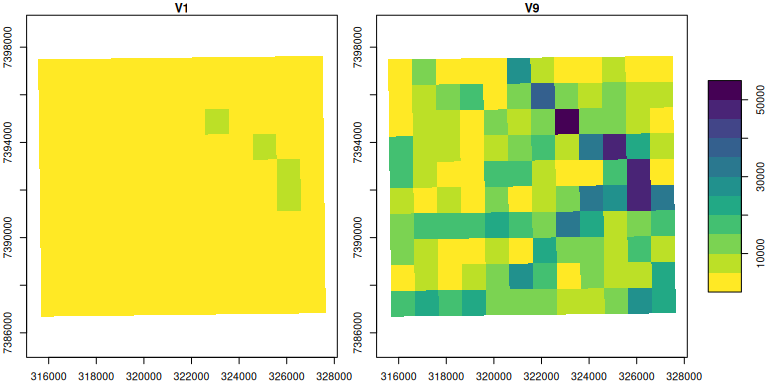#### Creating a WRFChem Input file using eixport:

1. Create a grid using make_grid and a wrfinput file
2. Run emis_grid to grid your emissions.
3. Create a GriddedEmissionsArray.
4. Create a wrfchem input file eixport :  : wrf_create(https://atmoschem.github.io/eixport/reference/wrf_create.html.
5. Put the GriddedEmissionsArray into the wrf chem input file using eixport::wrf_put.
library(eixport)
dir.create(file.path(tempdir(), "EMISS"))
wrf_create(wrfinput_dir         = system.file("extdata", package = "eixport"),
wrfchemi_dir         = file.path(tempdir(), "EMISS"),
domains              = 2,
frames_per_auxinput5 = 1, #hours
auxinput5_interval_m = 60,
verbose              = TRUE)
path_to_wrfi <- paste0(system.file("extdata", package = "eixport"), "/wrfinput_d02")
path_to_wrfc <- list.files(file.path(tempdir(), "EMISS"), full.names = TRUE)
gwrf <- eixport::wrf_grid(path_to_wrfi)
E_CO_gwrf <- emis_grid(spobj = E_CO_STREETSnet, g = gwrf)
gr <- GriddedEmissionsArray(E_CO_gwrf, rows = 51, cols = 63, times = 1)
eixport::wrf_put(file = path_to_wrfc, name = "E_CO", POL = gr)

#### Creating a WRFChem Input file using AS4WRF

1. Create a grid using make_grid and your net.
2. Run emis_grid to grid your emissions.
3. Run eixport::to_as4wrf to create a data.frame the specifications for AS4WRF.ncl.
4. Export data.frame to a text.file. Recall that AS4WRF requires all the lumped species.
5. Contact the developer of AS4WRF Angel Vara to get a copy and run AS4WRF.ncl.

Thanks and enjoy VEIN!

## Citation

If you use VEIN, please, cite it (BIBTEX, ENDNOTE):

Ibarra-Espinosa, S., Ynoue, R., O’Sullivan, S., Pebesma, E., Andrade, M. D. F., and Osses, M.: VEIN v0.2.2: an R package for bottom-up vehicular emissions inventories, Geosci. Model Dev., 11, 2209-2229, https://doi.org/10.5194/gmd-11-2209-2018, 2018.

@article{gmd-11-2209-2018,
author = {Ibarra-Espinosa, S. and Ynoue, R. and O'Sullivan, S. and Pebesma, E. and Andrade, M. D. F. and Osses, M.},
title = {VEIN v0.2.2: an R package for bottom--up vehicular emissions inventories},
journal = {Geoscientific Model Development},
volume = {11},
year = {2018},
number = {6},
pages = {2209--2229},
url = {https://gmd.copernicus.org/articles/11/2209/2018/},
doi = {10.5194/gmd-11-2209-2018}
}

## Issues

If you encounter any issues while using VEIN, please submit your issues to: https://github.com/atmoschem/vein/issues/ If you have any suggestions just let me know to .

### Contributing

Please, read this guide. Contributions of all sorts are welcome, issues and pull requests are the preferred ways of sharing them. When contributing pull requests, please follow the Google’s R Style Guide. This project is released with a Contributor Code of Conduct. By participating in this project you agree to abide by its terms.

### Note for non-english and anaconda users

Sometimes you need to install R and all dependencies and a way for doing that is using anaconda. Well, as my system is in portuguese, after installing R using anaconda it changed the decimal character to ‘,’. In order to change it back to english meaning decimal separator as ‘.’, I added this variable into the .bashrc

nano ~/.bashrc
export Lang=C

More details on StackOverflow